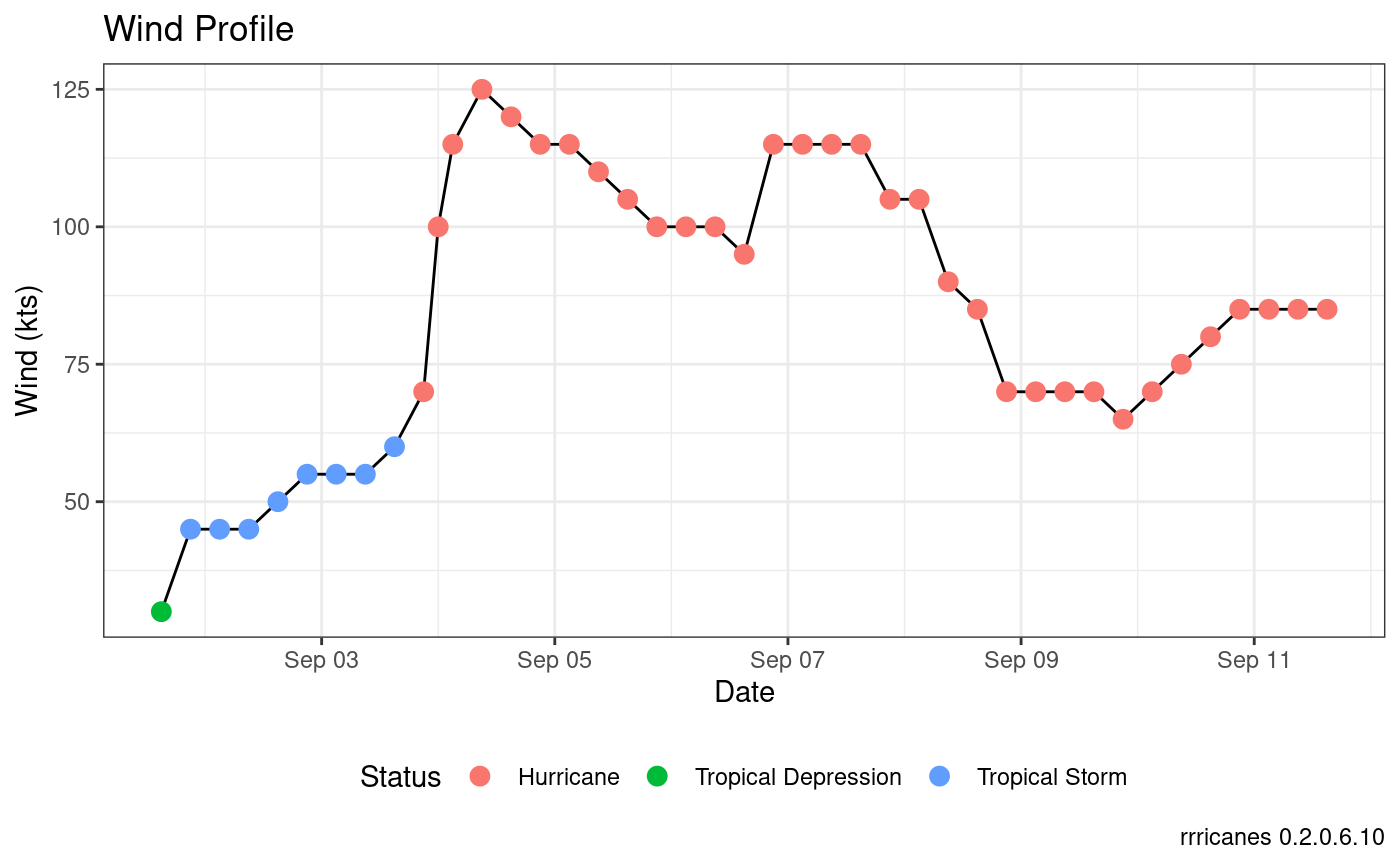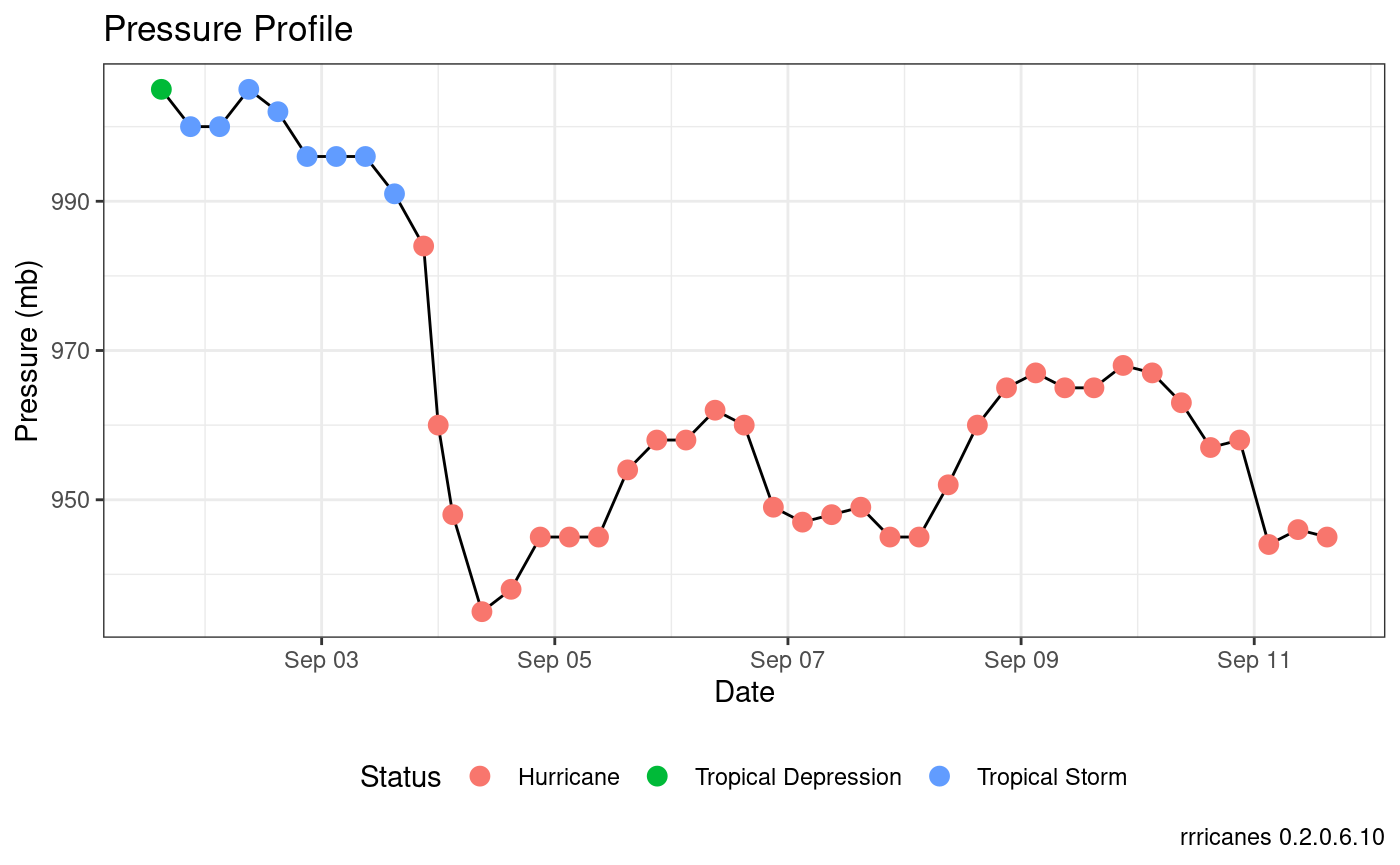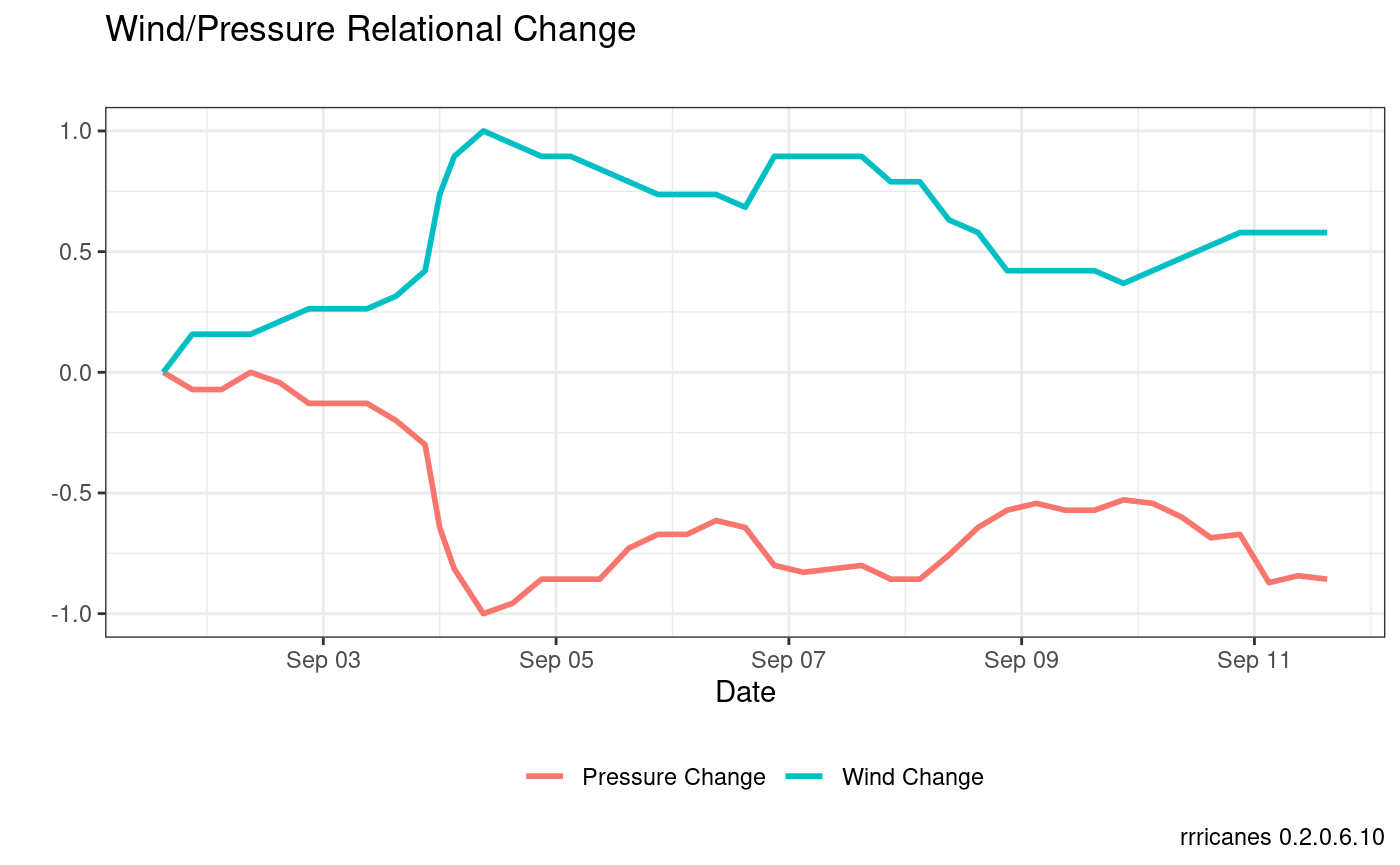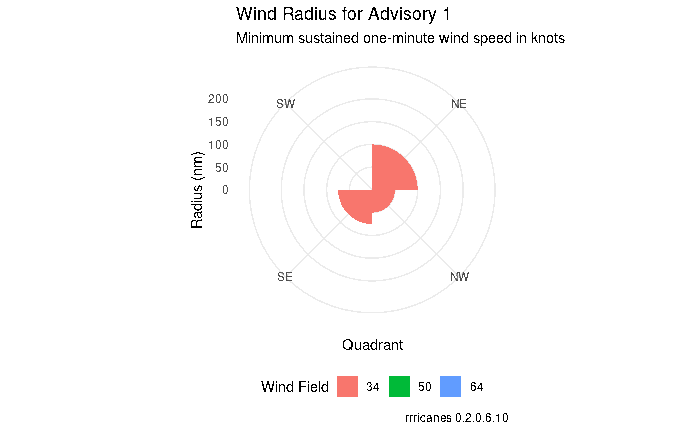``````library(dplyr)
library(gganimate)
library(ggplot2)
library(rrricanes)
library(rrricanesdata)
library(tidyr)``````
``````key <- "AL092008"

``fstadv <- fstadv %>% filter(Key == key, Adv <= adv)``

## Wind Profile

``````# Plot wind values
fstadv %>% ggplot(aes(x = Date, y = Wind)) +
geom_line() +
geom_point(aes(color = Status), size = 3) +
scale_y_continuous(name = "Wind (kts)") +
theme_bw() +
theme(legend.position = "bottom",
legend.box = "vertical") +
labs(title = "Wind Profile",
caption = sprintf("rrricanes %s", packageVersion("rrricanes")))``````## Pressure Profile

``````# Plot pressure values
fstadv %>% ggplot(aes(x = Date, y = Pressure)) +
geom_line() +
geom_point(aes(color = Status), size = 3) +
scale_y_continuous(name = "Pressure (mb)") +
theme_bw() +
theme(legend.position = "bottom",
legend.box = "vertical") +
labs(title = "Pressure Profile",
caption = sprintf("rrricanes %s", packageVersion("rrricanes")))``````## Wind/Pressure Relational Change

``````fstadv %>%
mutate(WindDist = (Wind - min(Wind))/(max(Wind) - min(Wind)),
PressDist = (Pressure - max(Pressure))/(max(Pressure) - min(Pressure))) %>%
gather(Var, Val, WindDist, PressDist) %>%
ggplot(aes(x = Date, y = Val, group = Var, color = Var)) +
geom_line(size = 1) +
scale_color_discrete(labels = c("Pressure Change", "Wind Change")) +
theme_bw() +
theme(legend.position = "bottom",
legend.title = element_blank()) +
labs(title = "Wind/Pressure Relational Change",
subtitle = "",
caption = sprintf("rrricanes %s", packageVersion("rrricanes")),
y = "")````````````wr_animate <-
tidy_wr() %>%
ggplot(
aes(
fill = factor(WindField),
)
) +
geom_bar(stat = "identity", position = "identity", width = 1) +
guides(fill = guide_legend(title = "Wind Field")) +
coord_polar() +
theme_minimal() +
theme(legend.position = "bottom") +
labs(
subtitle = "Minimum sustained one-minute wind speed in knots",
caption = sprintf("rrricanes %s", packageVersion("rrricanes")),